Home > ACC7 > Chapter cc27 > Lesson cc27.2.1 > Problem7-100

7-100.
1. Find the missing value in each of the pairs of equivalent ratios below. Homework Help ✎

1.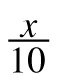=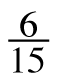2.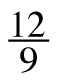=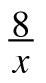3.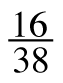=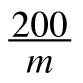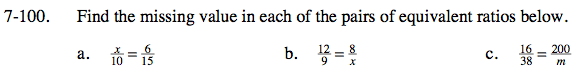Think about what values would go in a Giant One to turn tenths into fifteenths.

$\left(\frac{x}{10}\right)\left(\frac{1.5}{1.5}\right)=\frac{6}{15}$

x(1.5) = 6
What number multiplied by 1.5 gives 6?

x = 4

This problem is very similar to part (a).

x = 6

How could you use a Giant One to find the value of m?